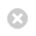Close

Mathematics

Home# Number Theory

There are several research groups at UCL working in algebraic number theory:

The research of Dokchitser and his team lies in the field of explicit arithmetic geometry, and primarily concerns the arithmetic of elliptic curves and of Jacobians of higher genus curves. It includes the study of L-values, the Birch-Swinnerton-Dyer conjecture, parity of ranks of Mordell-Weil groups, local and global Galois representations, and models and invariants of curves over local fields. While primarily focusing on theoretical results, their work is often aided by computational methods.

The research of García centres on the arithmetic and cohomology of locally symmetric spaces with a special focus on special cycles and relations with special values of L-functions. Locally symmetric spaces are manifolds that generalise the classical modular curves, and are of fundamental interest in number theory. Special cycles provide natural cohomology classes in these spaces that have been intensively studied in recent years, leading to the best results to date on central questions such as the Birch--Swinnerton-Dyer conjecture, the Stark conjectures, and their generalizations.

Hill and his research group are working on the cohomology of arithmetic groups, and in particular on Emerton’s p-adic Banach space representations. This theory fits into the wider framework of the (p-adic) Langlands programme, which is a very active and fast-developing area of research in number theory. The theory predicts a correspondence between certain classes of Banach space representations on the one hand and certain Galois representations on the other hand.

Yafaev and his group are interested in the properties of Shimura varieties. These varieties are generalisations of classical modular curves, and their geometry is the subject of a lot of current research. One of the central conjectures concerning Shimura varieties, which is studied by Yafaev and his group, is the Andre-Oort conjecture about the distribution of special points on the Shimura variety; for pure Shimura varieties, this conjecture was established by Yafaev and the French mathematician E. Ullmo under the Generalised Riemann Hypothesis.

The focus of Zerbes' research group is the arithmetic of modular and automorphic forms, with a particular interested in the special values of their L-functions. Special values of L-functions are a central topic in number theory: the most famous example is the Birch—Swinnerton-Dyer conjecture, which is one of the Clay Millennium Math Problems. One of the strongest results towards this conjecture was proved in the 1970s by the Russian mathematician V. Kolyvagin; the main tool in his work is a geometric construction called an Euler system. Zerbes’ research group is concerned with the construction of new Euler systems, with the aim of proving new cases of the Birch—Swinnerton-Dyer conjecture and its generalisations.

#### Members

Mr Jordan Docking (PhD Student)
Ms Holly Green (PhD Student)
Dr Chung-Hang Kwan (Postdoctoral Research Fellow)
Dr Caleb Springer (Postdoctoral Research Fellow)
Dr Alexander Walker (Postdoctoral Research Fellow)

### Analytic Number Theory

Petridis and his group are investigating analytic aspects of automorphic forms and, in particular, their spectral theory. The aims of such investigations include lattice point problems in hyperbolic space and, more general, affine homogeneous varieties, the distribution of modular symbols (conjectures of Mazur—Rubin, Goldfeld Eisenstein series), and relations with mathematical physics (quantum ergodicity of eigenfunctions of the Laplace operator). Central to such investigations is the analytic theory of L-functions e.g. the Riemann zeta function, and substitutes of the Generalized Riemann Hypothesis e.g. Lindelöf hypothesis, subconvexity estimates etc. The theory of exponential sums is also applied to the spectrum of Heisenberg manifolds, L-functions, and the Gauss circle problem. The whole theory has direct implications to correlations of arithmetic functions, e.g. sums of two squares.

Analytic number theory has traditionally developed through the examination of zeros of analytic continuations of very special functions (like the Riemann zeta function). Andrew Granville and Soundararajan have proposed an alternative approach (the "pretentious approach") which has become the source of several key developments in analytic number theory in the last few years. This approach is rather more general and combinatorial, allowing more flexibility. Granville's research group develops the broad picture of this approach: Bounds on moments of zeta, a multi-dimensional circle method, QUE in vanishing domains, structure of class groups and distribution of Selmer ranks, analytic number theory in function fields via Galois theory, properties of polynomial solutions to equations, the LSD method for averages.